## 线性回归和线性分类器¶

• 回归
• 线性分类
• 逻辑回归的正则化
• 逻辑回归的优缺点
• 验证和学习曲线

### 最小二乘法¶

$$y = w_0 + \sum_{i=1}^m w_i x_i$$

$$y = \sum_{i=0}^m w_i x_i = \textbf{w}^\text{T} \textbf{x}$$

$$\textbf y = \textbf X \textbf w + \epsilon$$

• $\textbf y \in \mathbb{R}^n$：因变量（目标变量）。
• $w$：模型的参数向量（在机器学习中，这些参数经常被称为权重）。
• $\textbf X$：观测及其特征矩阵，大小为 n 行、m+1 列（包括左侧的虚列），其秩的大小为 $\text{rank}\left(\textbf X\right) = m + 1$。
• $\epsilon$：一个变量，用来表示随机、不可预测模型的错误。

$$y_i = \sum_{j=0}^m w_j X_{ij} + \epsilon_i$$

• 随机误差的期望为零：$\forall i: \mathbb{E}\left[\epsilon_i\right] = 0$;
• 随机误差具有相同的有限方差，这一性质称为等分散性：$\forall i: \text{Var}\left(\epsilon_i\right) = \sigma^2 < \infty$;
• 随机误差不相关：$\forall i \neq j: \text{Cov}\left(\epsilon_i, \epsilon_j\right) = 0$.

$$\widehat{w}_i = \omega_{1i}y_1 + \omega_{2i}y_2 + \cdots + \omega_{ni}y_n$$

$$\mathbb{E}\left[\widehat{w}_i\right] = w_i$$

$$\begin{array}{rcl}\mathcal{L}\left(\textbf X, \textbf{y}, \textbf{w} \right) &=& \frac{1}{2n} \sum_{i=1}^n \left(y_i - \textbf{w}^\text{T} \textbf{x}_i\right)^2 \\ &=& \frac{1}{2n} \left\| \textbf{y} - \textbf X \textbf{w} \right\|_2^2 \\ &=& \frac{1}{2n} \left(\textbf{y} - \textbf X \textbf{w}\right)^\text{T} \left(\textbf{y} - \textbf X \textbf{w}\right) \end{array}$$

$$\begin{array}{rcl} \frac{\partial}{\partial \textbf{X}} \textbf{X}^{\text{T}} \textbf{A} &=& \textbf{A} \end{array}$$
$$\begin{array}{rcl} \frac{\partial}{\partial \textbf{X}} \textbf{X}^{\text{T}} \textbf{A} \textbf{X} &=& \left(\textbf{A} + \textbf{A}^{\text{T}}\right)\textbf{X} \end{array}$$
$$\begin{array}{rcl}\frac{\partial}{\partial \textbf{A}} \textbf{X}^{\text{T}} \textbf{A} \textbf{y} &=& \textbf{X}^{\text{T}} \textbf{y} \end{array}$$
$$\begin{array}{rcl} \frac{\partial}{\partial \textbf{X}} \textbf{A}^{-1} &=& -\textbf{A}^{-1} \frac{\partial \textbf{A}}{\partial \textbf{X}} \textbf{A}^{-1} \end{array}$$

$$\begin{array}{rcl} \frac{\partial \mathcal{L}}{\partial \textbf{w}} &=& \frac{\partial}{\partial \textbf{w}} \frac{1}{2n} \left( \textbf{y}^{\text{T}} \textbf{y} -2\textbf{y}^{\text{T}} \textbf{X} \textbf{w} + \textbf{w}^{\text{T}} \textbf{X}^{\text{T}} \textbf{X} \textbf{w}\right) \\ &=& \frac{1}{2n} \left(-2 \textbf{X}^{\text{T}} \textbf{y} + 2\textbf{X}^{\text{T}} \textbf{X} \textbf{w}\right) \end{array}$$
$$\begin{array}{rcl} \frac{\partial \mathcal{L}}{\partial \textbf{w}} = 0 &\Leftrightarrow& \frac{1}{2n} \left(-2 \textbf{X}^{\text{T}} \textbf{y} + 2\textbf{X}^{\text{T}} \textbf{X} \textbf{w}\right) = 0 \\ &\Leftrightarrow& -\textbf{X}^{\text{T}} \textbf{y} + \textbf{X}^{\text{T}} \textbf{X} \textbf{w} = 0 \\ &\Leftrightarrow& \textbf{X}^{\text{T}} \textbf{X} \textbf{w} = \textbf{X}^{\text{T}} \textbf{y} \\ &\Leftrightarrow& \textbf{w} = \left(\textbf{X}^{\text{T}} \textbf{X}\right)^{-1} \textbf{X}^{\text{T}} \textbf{y} \end{array}$$

### 最大似然估计¶

$$p\left(\theta, x\right) = \theta^x \left(1 - \theta\right)^\left(1 - x\right), x \in \left\{0, 1\right\}$$

$$p(\textbf{x}; \theta) = \prod_{i=1}^{400} \theta^{x_i} \left(1 - \theta\right)^{\left(1 - x_i\right)} = \theta^{117} \left(1 - \theta\right)^{283}$$

$$\log p(\textbf{x}; \theta) = \log \prod_{i=1}^{400} \theta^{x_i} \left(1 - \theta\right)^{\left(1 - x_i\right)} =$$
$$= \log \theta^{117} \left(1 - \theta\right)^{283} = 117 \log \theta + 283 \log \left(1 - \theta\right)$$

$$\frac{\partial \log p(\textbf{x}; \theta)}{\partial \theta} = \frac{\partial}{\partial \theta} \left(117 \log \theta + 283 \log \left(1 - \theta\right)\right) = \frac{117}{\theta} - \frac{283}{1 - \theta};$$

$$\textbf y = \textbf X \textbf w + \epsilon$$

$$\epsilon_i \sim \mathcal{N}\left(0, \sigma^2\right)$$

$$\begin{array}{rcl} y_i &=& \sum_{j=1}^m w_j X_{ij} + \epsilon_i \\ &\sim& \sum_{j=1}^m w_j X_{ij} + \mathcal{N}\left(0, \sigma^2\right) \\ p\left(y_i \mid \textbf X; \textbf{w}\right) &=& \mathcal{N}\left(\sum_{j=1}^m w_j X_{ij}, \sigma^2\right) \end{array}$$

$$\begin{array}{rcl} \log p\left(\textbf{y}\mid \textbf X; \textbf{w}\right) &=& \log \prod_{i=1}^n \mathcal{N}\left(\sum_{j=1}^m w_j X_{ij}, \sigma^2\right) \\ &=& \sum_{i=1}^n \log \mathcal{N}\left(\sum_{j=1}^m w_j X_{ij}, \sigma^2\right) \\ &=& -\frac{n}{2}\log 2\pi\sigma^2 -\frac{1}{2\sigma^2} \sum_{i=1}^n \left(y_i - \textbf{w}^\text{T} \textbf{x}_i\right)^2 \end{array}$$

$$\begin{array}{rcl} \textbf{w}_{\text{ML}} &=& \arg \max_{\textbf w} p\left(\textbf{y}\mid \textbf X; \textbf{w}\right) = \arg \max_{\textbf w} \log p\left(\textbf{y}\mid \textbf X; \textbf{w}\right)\\ &=& \arg \max_{\textbf w} -\frac{n}{2}\log 2\pi\sigma^2 -\frac{1}{2\sigma^2} \sum_{i=1}^n \left(y_i - \textbf{w}^{\text{T}} \textbf{x}_i\right)^2 \\ &=& \arg \max_{\textbf w} -\frac{1}{2\sigma^2} \sum_{i=1}^n \left(y_i - \textbf{w}^{\text{T}} \textbf{x}_i\right)^2 \\ &=& \arg \min_{\textbf w} \mathcal{L}\left(\textbf X, \textbf{y}, \textbf{w} \right) \end{array}$$

### 偏置-方差分解¶

• 目标变量的真值 $y$ 是确定性函数 $f\left(\textbf{x}\right)$ 和随机误差 $\epsilon$ 之和：$y = f\left(\textbf{x}\right) + \epsilon$。
• 误差符合均值为零、方差一致的正态分布：$\epsilon \sim \mathcal{N}\left(0, \sigma^2\right)$。
• 目标变量的真值亦为正态分布：$y \sim \mathcal{N}\left(f\left(\textbf{x}\right), \sigma^2\right)$。
• 试图使用一个协变量线性函数逼近一个未知的确定性函数 $f\left(\textbf{x}\right)$，这一协变量线性函数是函数空间中估计函数 $f$ 的一点，即均值和方差的随机变量。

$$\begin{array}{rcl} \text{Err}\left(\textbf{x}\right) &=& \mathbb{E}\left[\left(y - \widehat{f}\left(\textbf{x}\right)\right)^2\right] \\ &=& \mathbb{E}\left[y^2\right] + \mathbb{E}\left[\left(\widehat{f}\left(\textbf{x}\right)\right)^2\right] - 2\mathbb{E}\left[y\widehat{f}\left(\textbf{x}\right)\right] \\ &=& \mathbb{E}\left[y^2\right] + \mathbb{E}\left[\widehat{f}^2\right] - 2\mathbb{E}\left[y\widehat{f}\right] \\ \end{array}$$

$$\begin{array}{rcl} \mathbb{E}\left[y^2\right] &=& \text{Var}\left(y\right) + \mathbb{E}\left[y\right]^2 = \sigma^2 + f^2\\ \mathbb{E}\left[\widehat{f}^2\right] &=& \text{Var}\left(\widehat{f}\right) + \mathbb{E}\left[\widehat{f}\right]^2 \\ \end{array}$$

$$\begin{array}{rcl} \text{Var}\left(y\right) &=& \mathbb{E}\left[\left(y - \mathbb{E}\left[y\right]\right)^2\right] \\ &=& \mathbb{E}\left[\left(y - f\right)^2\right] \\ &=& \mathbb{E}\left[\left(f + \epsilon - f\right)^2\right] \\ &=& \mathbb{E}\left[\epsilon^2\right] = \sigma^2 \end{array}$$
$$\mathbb{E}[y] = \mathbb{E}[f + \epsilon] = \mathbb{E}[f] + \mathbb{E}[\epsilon] = f$$

$$\begin{array}{rcl} \mathbb{E}\left[y\widehat{f}\right] &=& \mathbb{E}\left[\left(f + \epsilon\right)\widehat{f}\right] \\ &=& \mathbb{E}\left[f\widehat{f}\right] + \mathbb{E}\left[\epsilon\widehat{f}\right] \\ &=& f\mathbb{E}\left[\widehat{f}\right] + \mathbb{E}\left[\epsilon\right] \mathbb{E}\left[\widehat{f}\right] = f\mathbb{E}\left[\widehat{f}\right] \end{array}$$

$$\begin{array}{rcl} \text{Err}\left(\textbf{x}\right) &=& \mathbb{E}\left[\left(y - \widehat{f}\left(\textbf{x}\right)\right)^2\right] \\ &=& \sigma^2 + f^2 + \text{Var}\left(\widehat{f}\right) + \mathbb{E}\left[\widehat{f}\right]^2 - 2f\mathbb{E}\left[\widehat{f}\right] \\ &=& \left(f - \mathbb{E}\left[\widehat{f}\right]\right)^2 + \text{Var}\left(\widehat{f}\right) + \sigma^2 \\ &=& \text{Bias}\left(\widehat{f}\right)^2 + \text{Var}\left(\widehat{f}\right) + \sigma^2 \end{array}$$

• 偏差（bias）: $\text{Bias}\left(\widehat{f}\right)$ 度量了学习算法的期望输出与真实结果的偏离程度, 刻画了算法的拟合能力，偏差偏高表示预测函数与真实结果差异很大。
• 方差（variance）: $\text{Var}\left(\widehat{f}\right)$ 代表「同样大小的不同的训练数据集训练出的模型」与「这些模型的期望输出值」之间的差异。训练集变化导致性能变化，方差偏高表示模型很不稳定。
• 不可消除的误差（irremovable error）: $\sigma^2$ 刻画了当前任务任何算法所能达到的期望泛化误差的下界，即刻画了问题本身的难度。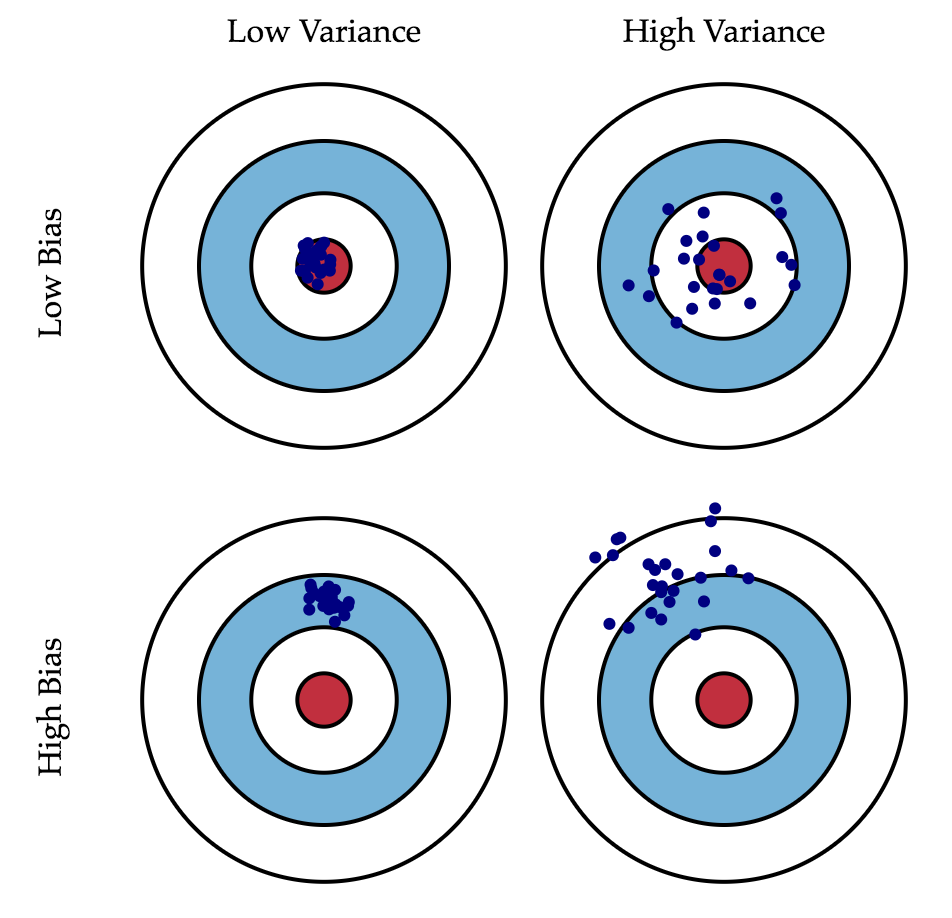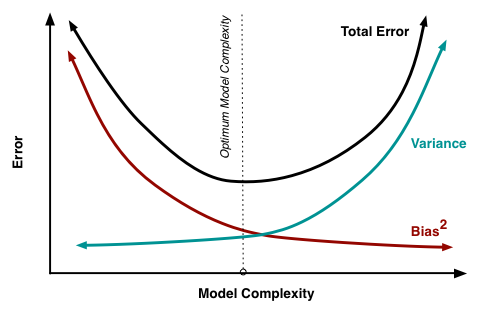### 线性回归的正则化¶

$$\begin{array}{rcl} \mathcal{L}\left(\textbf{X}, \textbf{y}, \textbf{w} \right) &=& \frac{1}{2n} \left\| \textbf{y} - \textbf{X} \textbf{w} \right\|_2^2 + \left\| \Gamma \textbf{w}\right\|^2\end{array}$$

$$\begin{array}{rcl} \textbf{w} &=& \left(\textbf{X}^{\text{T}} \textbf{X} + \lambda \textbf{E}\right)^{-1} \textbf{X}^{\text{T}} \textbf{y} \end{array}$$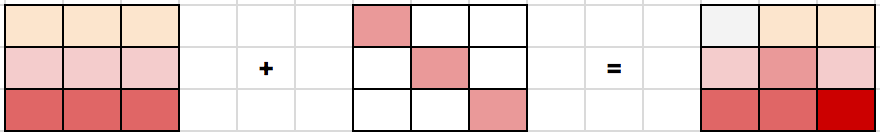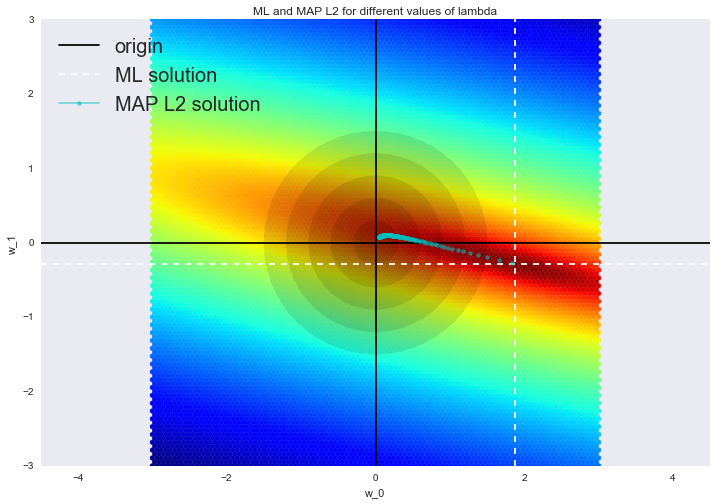### 线性分类¶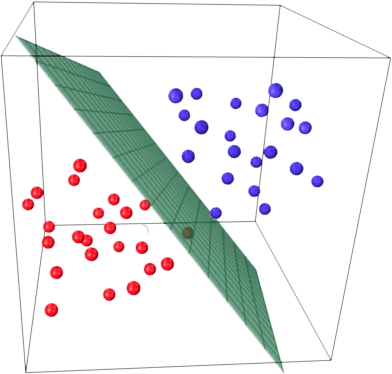$$a(\textbf{x}) = \text{sign}(\textbf{w}^\text{T}\textbf x)$$

• $\textbf{x}$ 是特征向量（包括标识）。
• $\textbf{w}$ 是线性模型中的权重向量（偏置为 $w_0$）。
• $\text{sign}(\bullet)$ 是符号函数，返回参数的符号。
• $a(\textbf{x})$ 是分类 $\textbf{x}$ 的分类器。

### 基于逻辑回归的线性分类器¶

$$p_+ = P\left(y_i = 1 \mid \textbf{x}_\text{i}, \textbf{w}\right)$$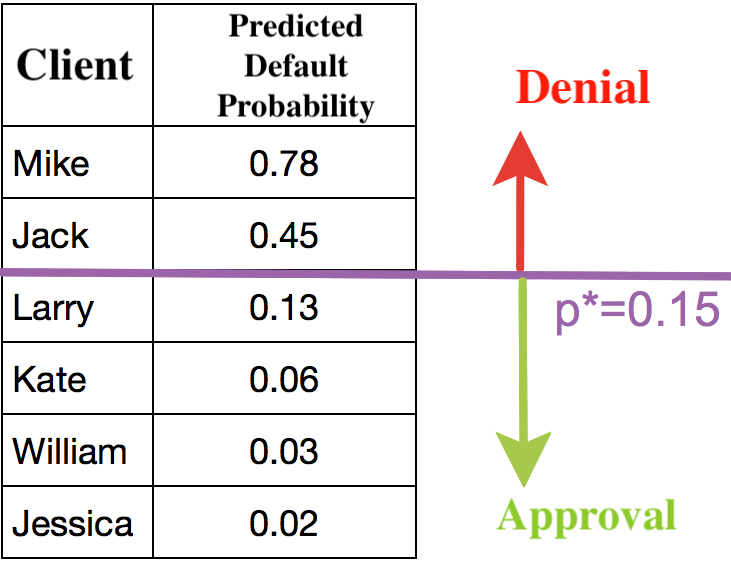$$b(\textbf{x}) = \textbf{w}^\text{T} \textbf{x} \in \mathbb{R}$$

$$\sigma(z) = \frac{1}{1 + \exp^{-z}}$$

In :
import warnings

import numpy as np
import seaborn as sns
from matplotlib import pyplot as plt

%matplotlib inline
warnings.filterwarnings("ignore")

In :
def sigma(z):
return 1.0 / (1 + np.exp(-z))

xx = np.linspace(-10, 10, 1000)
plt.plot(xx, [sigma(x) for x in xx])
plt.xlabel("z")
plt.ylabel("sigmoid(z)")
plt.title("Sigmoid function")

Out:
Text(0.5, 1.0, 'Sigmoid function')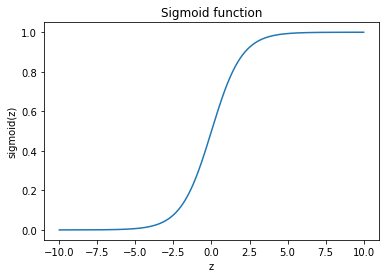$$p_+ = P\left(y_i = 1 \mid \textbf{x}_\text{i}, \textbf{w}\right)$$

$$w_{0}+w_{1}x_1 + w_{2}x_2 + ... = \textbf{w}^\text{T}\textbf{x}$$

$$\log(OR_{+}) = \textbf{w}^\text{T}\textbf{x}$$

$$p_{+} = \frac{OR_{+}}{1 + OR_{+}} = \frac{\exp^{\textbf{w}^\text{T}\textbf{x}}}{1 + \exp^{\textbf{w}^\text{T}\textbf{x}}} = \frac{1}{1 + \exp^{-\textbf{w}^\text{T}\textbf{x}}} = \sigma(\textbf{w}^\text{T}\textbf{x})$$

$$p_+(\textbf{x}_\text{i}) = P\left(y_i = 1 \mid \textbf{x}_\text{i}, \textbf{w}\right) = \sigma(\textbf{w}^\text{T}\textbf{x}_\text{i}).$$

### 最大似然估计和逻辑回归¶

$$p_+(\textbf{x}_\text{i}) = P\left(y_i = 1 \mid \textbf{x}_\text{i}, \textbf{w}\right) = \sigma(\textbf{w}^T\textbf{x}_\text{i})$$

「-」分类相应的表达式为：

$$p_-(\textbf{x}_\text{i}) = P\left(y_i = -1 \mid \textbf{x}_\text{i}, \textbf{w}\right) = 1 - \sigma(\textbf{w}^T\textbf{x}_\text{i}) = \sigma(-\textbf{w}^T\textbf{x}_\text{i})$$

$$P\left(y = y_i \mid \textbf{x}_\text{i}, \textbf{w}\right) = \sigma(y_i\textbf{w}^T\textbf{x}_\text{i})$$

$$\rho(\textbf{x}_A, \textbf{w}^\text{T}\textbf{x} = 0) = \frac{\textbf{w}^\text{T}\textbf{x}_A}{||\textbf{w}||}$$• 如果边缘的绝对值较大，且为正值，那么分类的标签是正确的，且目标离分界超平面很远，也就是模型对这一分类很肯定。如下图点 $x_3$ 所示。
• 如果边缘的绝对值较大，且为负值，那么分类的标签是错误的，且目标离分界超平面很远，那么目标很可能是一个异常值（例如，它可能为训练集中一个错误标记的值）。如下图点 $x_1$ 所示。
• 如果边缘绝对值较小，那么目标距离分界超平面很近，其符号就决定了目标「是否被正确分类」。如下图点 $x_2$ 和 $x_4$ 所示。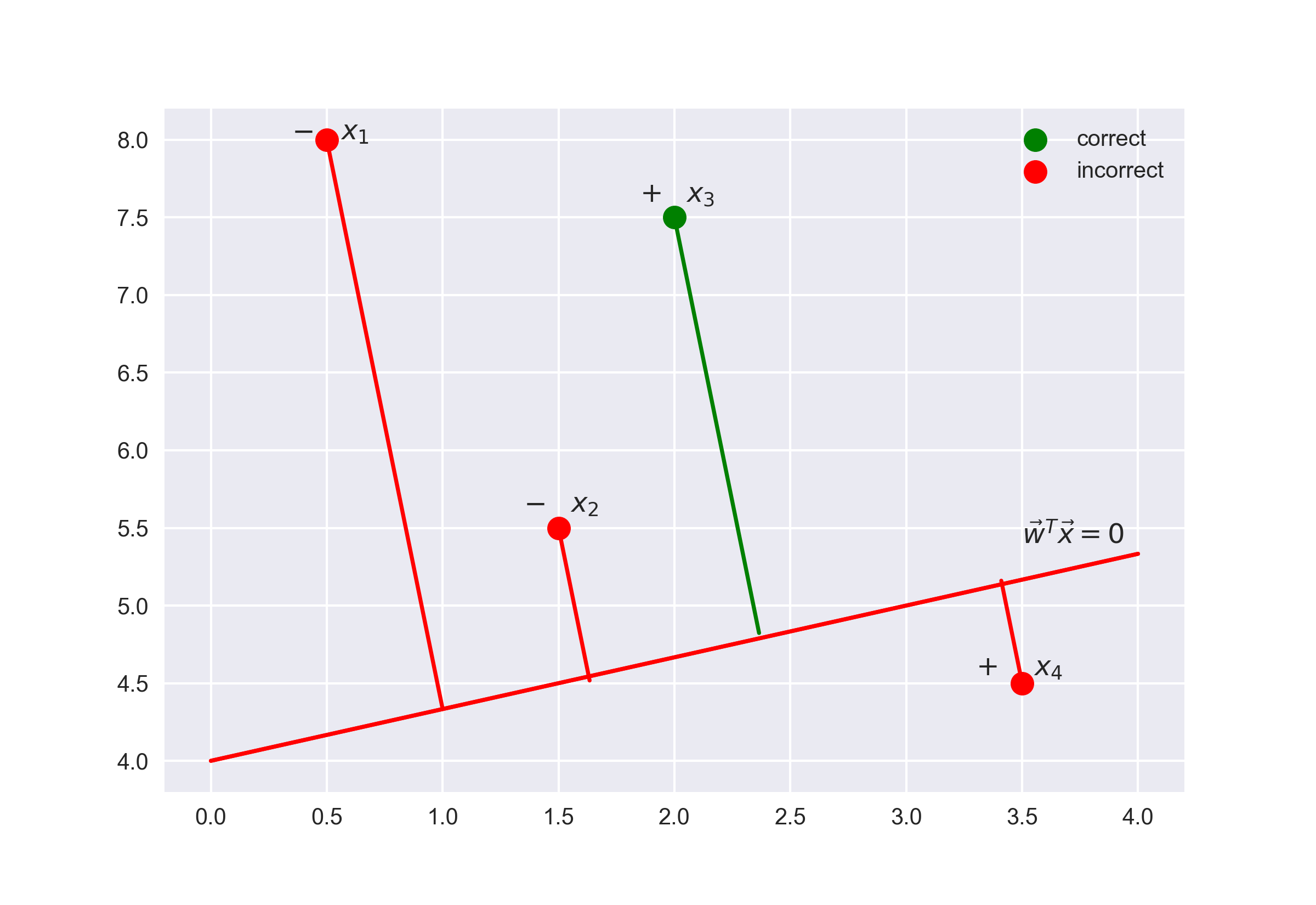$$P\left(\textbf{y} \mid \textbf{X}, \textbf{w}\right) = \prod_{i=1}^{\ell} P\left(y = y_i \mid \textbf{x}_\text{i}, \textbf{w}\right),$$

$$\log P\left(\textbf{y} \mid \textbf{X}, \textbf{w}\right) = \log \prod_{i=1}^{\ell} P\left(y = y_i \mid \textbf{x}_\text{i}, \textbf{w}\right) = \log \prod_{i=1}^{\ell} \sigma(y_i\textbf{w}^\text{T}\textbf{x}_\text{i}) =$$
$$= \sum_{i=1}^{\ell} \log \sigma(y_i\textbf{w}^\text{T}\textbf{x}_\text{i}) = \sum_{i=1}^{\ell} \log \frac{1}{1 + \exp^{-y_i\textbf{w}^\text{T}\textbf{x}_\text{i}}} = - \sum_{i=1}^{\ell} \log (1 + \exp^{-y_i\textbf{w}^\text{T}\textbf{x}_\text{i}})$$

$$\mathcal{L_{\log}} (\textbf X, \textbf{y}, \textbf{w}) = \sum_{i=1}^{\ell} \log (1 + \exp^{-y_i\textbf{w}^\text{T}\textbf{x}_\text{i}}).$$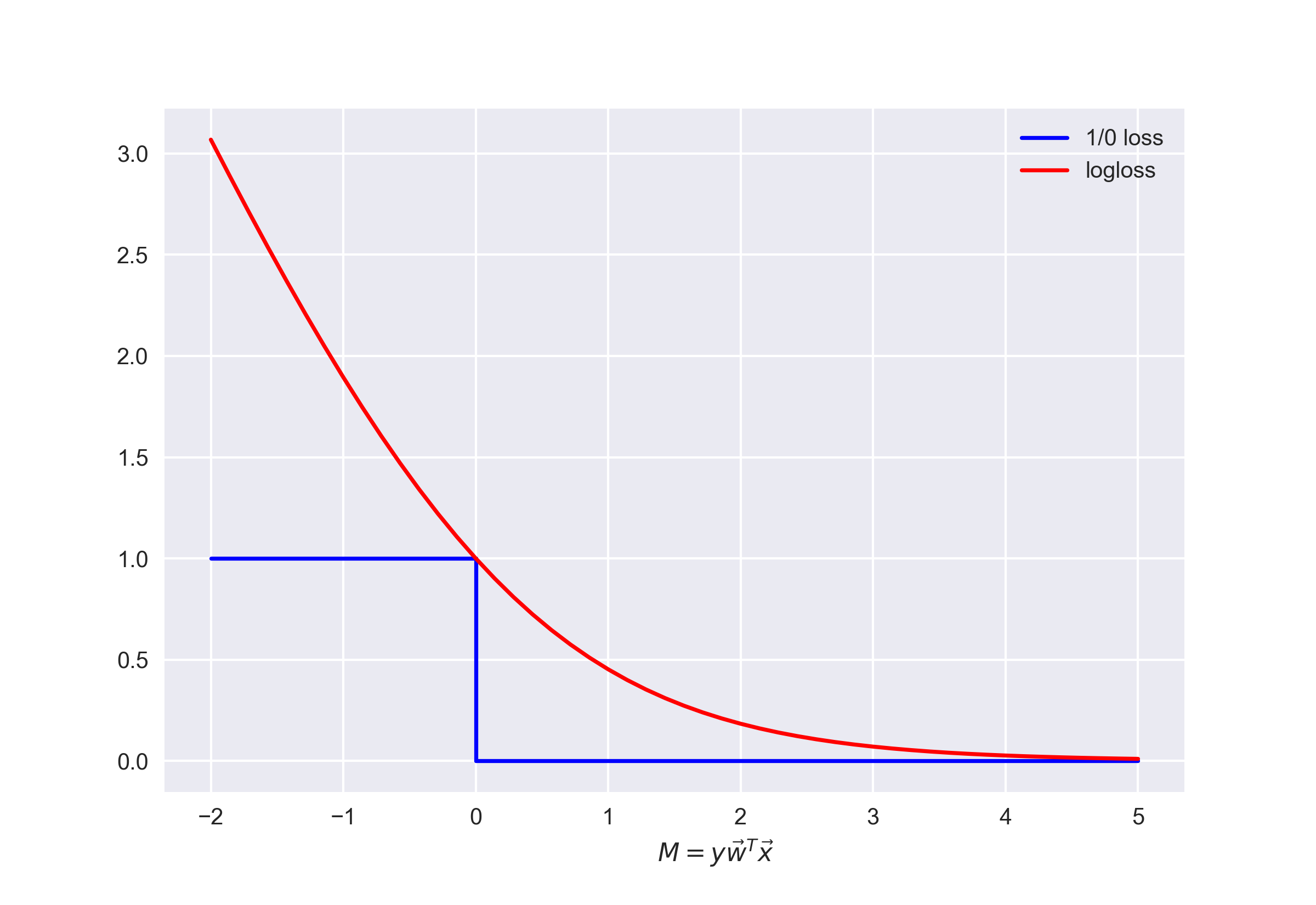$$\mathcal{L_{1/0}} (\textbf X, \textbf{y}, \textbf{w}) = \sum_{i=1}^{\ell} [M(\textbf{x}_\text{i}) < 0] \leq \sum_{i=1}^{\ell} \log (1 + \exp^{-y_i\textbf{w}^\text{T}\textbf{x}_\text{i}}) = \mathcal{L_{\log}} (\textbf X, \textbf{y}, \textbf{w}),$$

### 逻辑回归的 $L_2$ 正则化¶

$$\mathcal{J}(\textbf X, \textbf{y}, \textbf{w}) = \mathcal{L_{\log}} (\textbf X, \textbf{y}, \textbf{w}) + \lambda |\textbf{w}|^2$$

$$\widehat{\textbf w} = \arg \min_{\textbf{w}} \mathcal{J}(\textbf X, \textbf{y}, \textbf{w}) = \arg \min_{\textbf{w}}\ (C\sum_{i=1}^{\ell} \log (1 + \exp^{-y_i\textbf{w}^\text{T}\textbf{x}_\text{i}})+ |\textbf{w}|^2)$$

### 逻辑回归正则化示例¶

In :
import numpy as np
import pandas as pd
import seaborn as sns
from matplotlib import pyplot as plt
from sklearn.linear_model import LogisticRegression, LogisticRegressionCV
from sklearn.model_selection import (GridSearchCV, StratifiedKFold,
cross_val_score)
from sklearn.preprocessing import PolynomialFeatures

%matplotlib inline


In :
# 读取数据集
)
# 查看数据集的一些信息
data.info()

<class 'pandas.core.frame.DataFrame'>
RangeIndex: 118 entries, 0 to 117
Data columns (total 3 columns):
test1       118 non-null float64
test2       118 non-null float64
released    118 non-null int64
dtypes: float64(2), int64(1)
memory usage: 2.8 KB


In :
data.head(5)

Out:
test1 test2 released
0 0.051267 0.69956 1
1 -0.092742 0.68494 1
2 -0.213710 0.69225 1
3 -0.375000 0.50219 1
4 -0.513250 0.46564 1
In :
data.tail(5)

Out:
test1 test2 released
113 -0.720620 0.538740 0
114 -0.593890 0.494880 0
115 -0.484450 0.999270 0
116 -0.006336 0.999270 0
117 0.632650 -0.030612 0

In :
X = data.iloc[:, :2].values
y = data.iloc[:, 2].values


In :
plt.scatter(X[y == 1, 0], X[y == 1, 1], c="blue", label="Released")
plt.scatter(X[y == 0, 0], X[y == 0, 1], c="orange", label="Faulty")
plt.xlabel("Test 1")
plt.ylabel("Test 2")
plt.title("2 tests of microchips. Logit with C=1")
plt.legend()

Out:
<matplotlib.legend.Legend at 0x7fd917ecc2e8>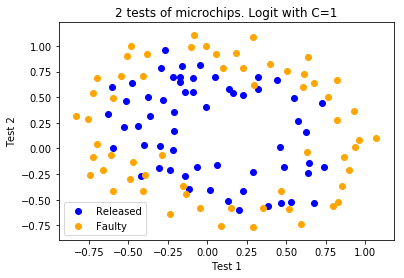In :
def plot_boundary(clf, X, y, grid_step=0.01, poly_featurizer=None):
x_min, x_max = X[:, 0].min() - 0.1, X[:, 0].max() + 0.1
y_min, y_max = X[:, 1].min() - 0.1, X[:, 1].max() + 0.1
xx, yy = np.meshgrid(
np.arange(x_min, x_max, grid_step), np.arange(y_min, y_max, grid_step)
)

# 在 [x_min, m_max]x[y_min, y_max] 的每一点都用它自己的颜色来对应
Z = clf.predict(poly_featurizer.transform(np.c_[xx.ravel(), yy.ravel()]))
Z = Z.reshape(xx.shape)
plt.contour(xx, yy, Z, cmap=plt.cm.Paired)


$$\{x_1^d, x_1^{d-1}x_2, \ldots x_2^d\} = \{x_1^ix_2^j\}_{i+j=d, i,j \in \mathbb{N}}$$

$$1, x_1, x_2, x_1^2, x_1x_2, x_2^2, x_1^3, x_1^2x_2, x_1x_2^2, x_2^3$$

In :
poly = PolynomialFeatures(degree=7)
X_poly = poly.fit_transform(X)

In :
X_poly.shape

Out:
(118, 36)

In :
C = 1e-2
logit = LogisticRegression(C=C, random_state=17)
logit.fit(X_poly, y)

plot_boundary(logit, X, y, grid_step=0.01, poly_featurizer=poly)

plt.scatter(X[y == 1, 0], X[y == 1, 1], c="blue", label="Released")
plt.scatter(X[y == 0, 0], X[y == 0, 1], c="orange", label="Faulty")
plt.xlabel("Test 1")
plt.ylabel("Test 2")
plt.title("2 tests of microchips. Logit with C=%s" % C)
plt.legend()

print("Accuracy on training set:", round(logit.score(X_poly, y), 3))

Accuracy on training set: 0.627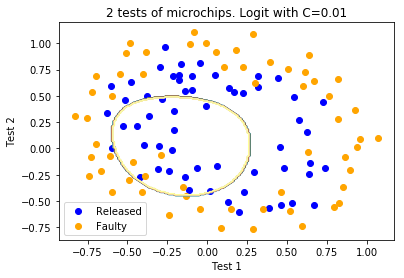In :
C = 1
logit = LogisticRegression(C=C, random_state=17)
logit.fit(X_poly, y)

plot_boundary(logit, X, y, grid_step=0.005, poly_featurizer=poly)

plt.scatter(X[y == 1, 0], X[y == 1, 1], c="blue", label="Released")
plt.scatter(X[y == 0, 0], X[y == 0, 1], c="orange", label="Faulty")
plt.xlabel("Test 1")
plt.ylabel("Test 2")
plt.title("2 tests of microchips. Logit with C=%s" % C)
plt.legend()

print("Accuracy on training set:", round(logit.score(X_poly, y), 3))

Accuracy on training set: 0.831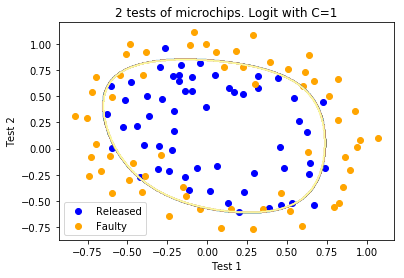In :
C = 1e4
logit = LogisticRegression(C=C, random_state=17)
logit.fit(X_poly, y)

plot_boundary(logit, X, y, grid_step=0.005, poly_featurizer=poly)

plt.scatter(X[y == 1, 0], X[y == 1, 1], c="blue", label="Released")
plt.scatter(X[y == 0, 0], X[y == 0, 1], c="orange", label="Faulty")
plt.xlabel("Test 1")
plt.ylabel("Test 2")
plt.title("2 tests of microchips. Logit with C=%s" % C)
plt.legend()

print("Accuracy on training set:", round(logit.score(X_poly, y), 3))

Accuracy on training set: 0.873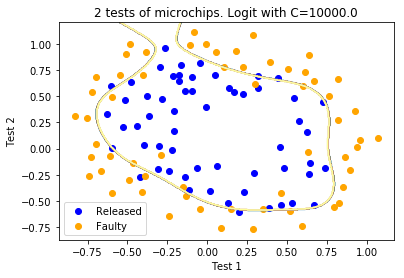$$J(X,y,w) = \mathcal{L} + \frac{1}{C}||w||^2,$$

• $\mathcal{L}$ 是对整个数据集的总逻辑损失函数
• $C$ 是反向正则化系数

• 参数 $C$ 越大，模型中可恢复的数据之间的关系就越复杂（直观地说，$C$ 对应模型的「复杂度」：模型能力）。
• 如果正则化过强，即 $C$ 值很小，最小化逻辑损失函数问题的解（权重）可能过小或为零。这样的模型对误差的「惩罚」也不够（即在上面的函数 $J(X,y,w)$ 中，权重的平方和过高，导致误差 $L$ 较大）。在这一情形下，模型将会欠拟合。
• 如果正则化过弱，即 $C$ 值很大，最小化逻辑损失函数问题的解可能权重过大。在这一情形下， $L$ 对函数 $J(X,y,w)$ 贡献较大，导致过拟合。
• $C$ 是一个超参数，逻辑回归不会自动学习调整 $C$ 的值，我们可以通过交叉验证来人工选择较好的 $C$ 值。

### 正则化参数的调整¶

In :
# 该单元格执行时间较长，请耐心等待
skf = StratifiedKFold(n_splits=5, shuffle=True, random_state=17)
# 下方结尾的切片为了在线上环境搜索更快，线下练习时可以删除
c_values = np.logspace(-2, 3, 500)[50:450:50]

logit_searcher = LogisticRegressionCV(Cs=c_values, cv=skf, verbose=1, n_jobs=-1)
logit_searcher.fit(X_poly, y)

[Parallel(n_jobs=-1)]: Using backend LokyBackend with 2 concurrent workers.
[Parallel(n_jobs=-1)]: Done   5 out of   5 | elapsed:    5.1s finished

Out:
LogisticRegressionCV(Cs=array([3.16958e-02, 1.00463e-01, 3.18424e-01, 1.00927e+00, 3.19897e+00,
1.01394e+01, 3.21376e+01, 1.01863e+02]),
class_weight=None,
cv=StratifiedKFold(n_splits=5, random_state=17, shuffle=True),
dual=False, fit_intercept=True, intercept_scaling=1.0,
max_iter=100, multi_class='warn', n_jobs=-1, penalty='l2',
random_state=None, refit=True, scoring=None, solver='lbfgs',
tol=0.0001, verbose=1)
In :
logit_searcher.C_

Out:
array([10.13939458])

In :
plt.plot(c_values, np.mean(logit_searcher.scores_, axis=0))
plt.xlabel("C")
plt.ylabel("Mean CV-accuracy")

Out:
Text(0, 0.5, 'Mean CV-accuracy')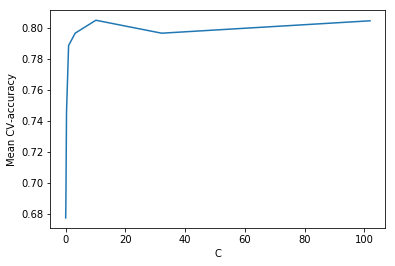In :
plt.plot(c_values, np.mean(logit_searcher.scores_, axis=0))
plt.xlabel("C")
plt.ylabel("Mean CV-accuracy")
plt.xlim((0, 10))

Out:
(0, 10)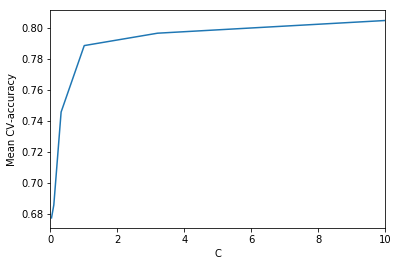### 逻辑回归的优缺点¶

#### 分析 IMDB 二元分类问题¶

IMDB 数据集中的训练集包含标记好的影评，其中有 12500 条好评，12500 条差评。使用词袋模型构建输入矩阵 $X$ ，语料库包含所有用户影评，影评的特征将由整个语料库中每个词的出现情况来表示。下图展示了这一思路：In :
import os

import matplotlib.pyplot as plt
import numpy as np
from sklearn.feature_extraction.text import CountVectorizer
from sklearn.linear_model import LogisticRegression


In [ ]:
# 文件较多，请耐心等待解压完成
!wget -nc "../../data/aclImdb_v1.tar.gz"
!tar -zxvf "aclImdb_v1.tar.gz"

In :
PATH_TO_IMDB = "aclImdb/"
os.path.join(PATH_TO_IMDB, "train"), categories=["pos", "neg"]
)
text_train, y_train = reviews_train.data, reviews_train.target
reviews_test = load_files(os.path.join(PATH_TO_IMDB, "test"), categories=["pos", "neg"])
text_test, y_test = reviews_test.data, reviews_test.target


In :
print("Number of documents in training data: %d" % len(text_train))
print(np.bincount(y_train))
print("Number of documents in test data: %d" % len(text_test))
print(np.bincount(y_test))

Number of documents in training data: 25000
[12500 12500]
Number of documents in test data: 25000
[12500 12500]


In :
text_train

Out:
b'Words can\'t describe how bad this movie is. I can\'t explain it by writing only. You have too see it for yourself to get at grip of how horrible a movie really can be. Not that I recommend you to do that. There are so many clich\xc3\xa9s, mistakes (and all other negative things you can imagine) here that will just make you cry. To start with the technical first, there are a LOT of mistakes regarding the airplane. I won\'t list them here, but just mention the coloring of the plane. They didn\'t even manage to show an airliner in the colors of a fictional airline, but instead used a 747 painted in the original Boeing livery. Very bad. The plot is stupid and has been done many times before, only much, much better. There are so many ridiculous moments here that i lost count of it really early. Also, I was on the bad guys\' side all the time in the movie, because the good guys were so stupid. "Executive Decision" should without a doubt be you\'re choice over this one, even the "Turbulence"-movies are better. In fact, every other movie in the world is better than this one.'

In :
y_train

Out:
0

y_train=0 表示影评是差评，y_train=1 表示影评是好评，上面这条影片是差评。

#### 单词的简单计数¶

In :
cv = CountVectorizer()
cv.fit(text_train)

len(cv.vocabulary_)

Out:
74849

In :
print(cv.get_feature_names()[:50])
print(cv.get_feature_names()[50000:50050])

['00', '000', '0000000000001', '00001', '00015', '000s', '001', '003830', '006', '007', '0079', '0080', '0083', '0093638', '00am', '00pm', '00s', '01', '01pm', '02', '020410', '029', '03', '04', '041', '05', '050', '06', '06th', '07', '08', '087', '089', '08th', '09', '0f', '0ne', '0r', '0s', '10', '100', '1000', '1000000', '10000000000000', '1000lb', '1000s', '1001', '100b', '100k', '100m']
['pincher', 'pinchers', 'pinches', 'pinching', 'pinchot', 'pinciotti', 'pine', 'pineal', 'pineapple', 'pineapples', 'pines', 'pinet', 'pinetrees', 'pineyro', 'pinfall', 'pinfold', 'ping', 'pingo', 'pinhead', 'pinheads', 'pinho', 'pining', 'pinjar', 'pink', 'pinkerton', 'pinkett', 'pinkie', 'pinkins', 'pinkish', 'pinko', 'pinks', 'pinku', 'pinkus', 'pinky', 'pinnacle', 'pinnacles', 'pinned', 'pinning', 'pinnings', 'pinnochio', 'pinnocioesque', 'pino', 'pinocchio', 'pinochet', 'pinochets', 'pinoy', 'pinpoint', 'pinpoints', 'pins', 'pinsent']


In :
X_train = cv.transform(text_train)
X_train

Out:
<25000x74849 sparse matrix of type '<class 'numpy.int64'>'
with 3445861 stored elements in Compressed Sparse Row format>

In :
text_train

Out:
b'This movie is terrible but it has some good effects.'

In :
X_train.nonzero()

Out:
array([ 9881, 21020, 28068, 29999, 34585, 34683, 44147, 61617, 66150,
66562], dtype=int32)
In :
X_train.nonzero()

Out:
(array([0, 0, 0, 0, 0, 0, 0, 0, 0, 0], dtype=int32),
array([ 9881, 21020, 28068, 29999, 34585, 34683, 44147, 61617, 66150,
66562], dtype=int32))

In :
X_test = cv.transform(text_test)


In :
logit = LogisticRegression(solver="lbfgs", n_jobs=-1, random_state=7)
logit.fit(X_train, y_train)

Out:
LogisticRegression(C=1.0, class_weight=None, dual=False, fit_intercept=True,
intercept_scaling=1, max_iter=100, multi_class='warn', n_jobs=-1,
penalty='l2', random_state=7, solver='lbfgs', tol=0.0001,
verbose=0, warm_start=False)

In :
round(logit.score(X_train, y_train), 3), round(logit.score(X_test, y_test), 3),

Out:
(0.981, 0.864)

In :
def visualize_coefficients(classifier, feature_names, n_top_features=25):
# get coefficients with large absolute values
coef = classifier.coef_.ravel()
positive_coefficients = np.argsort(coef)[-n_top_features:]
negative_coefficients = np.argsort(coef)[:n_top_features]
interesting_coefficients = np.hstack([negative_coefficients, positive_coefficients])
# plot them
plt.figure(figsize=(15, 5))
colors = ["red" if c < 0 else "blue" for c in coef[interesting_coefficients]]
plt.bar(np.arange(2 * n_top_features), coef[interesting_coefficients], color=colors)
feature_names = np.array(feature_names)
plt.xticks(
np.arange(1, 1 + 2 * n_top_features),
feature_names[interesting_coefficients],
rotation=60,
ha="right",
)

In :
def plot_grid_scores(grid, param_name):
plt.plot(
grid.param_grid[param_name],
grid.cv_results_["mean_train_score"],
color="green",
label="train",
)
plt.plot(
grid.param_grid[param_name],
grid.cv_results_["mean_test_score"],
color="red",
label="test",
)
plt.legend()

In :
visualize_coefficients(logit, cv.get_feature_names())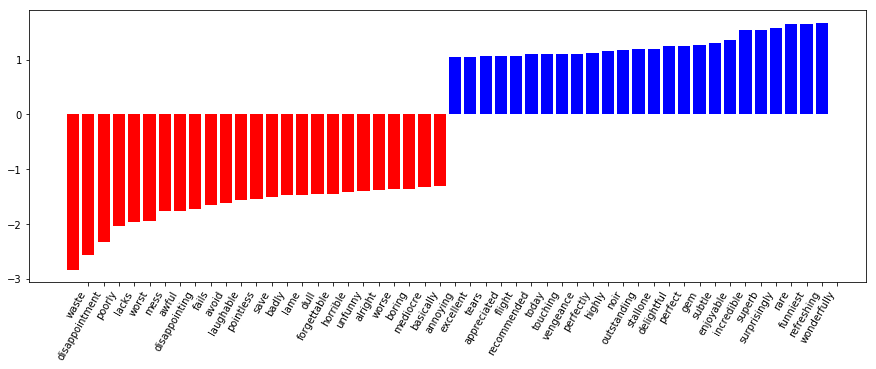In :
from sklearn.pipeline import make_pipeline

# 该单元格执行时间较长，请耐心等待
text_pipe_logit = make_pipeline(
CountVectorizer(), LogisticRegression(solver="lbfgs", n_jobs=1, random_state=7)
)

text_pipe_logit.fit(text_train, y_train)
print(text_pipe_logit.score(text_test, y_test))

0.864

In :
from sklearn.model_selection import GridSearchCV

# 该单元格执行时间较长，请耐心等待
param_grid_logit = {"logisticregression__C": np.logspace(-5, 0, 6)[4:5]}
grid_logit = GridSearchCV(
text_pipe_logit, param_grid_logit, return_train_score=True, cv=3, n_jobs=-1
)

grid_logit.fit(text_train, y_train)

Out:
GridSearchCV(cv=3, error_score='raise-deprecating',
estimator=Pipeline(memory=None,
steps=[('countvectorizer', CountVectorizer(analyzer='word', binary=False, decode_error='strict',
dtype=<class 'numpy.int64'>, encoding='utf-8', input='content',
lowercase=True, max_df=1.0, max_features=None, min_df=1,
ngram_range=(1, 1), preprocessor=None, stop_words=None,
...  penalty='l2', random_state=7, solver='lbfgs', tol=0.0001,
verbose=0, warm_start=False))]),
fit_params=None, iid='warn', n_jobs=-1,
param_grid={'logisticregression__C': array([0.1])},
pre_dispatch='2*n_jobs', refit=True, return_train_score=True,
scoring=None, verbose=0)

In :
grid_logit.best_params_, grid_logit.best_score_

Out:
({'logisticregression__C': 0.1}, 0.8848)
In :
plot_grid_scores(grid_logit, "logisticregression__C")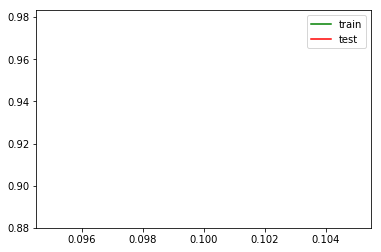In :
grid_logit.score(text_test, y_test)

Out:
0.87812

In :
from sklearn.ensemble import RandomForestClassifier

forest = RandomForestClassifier(n_estimators=200, n_jobs=-1, random_state=17)

In :
forest.fit(X_train, y_train)

Out:
RandomForestClassifier(bootstrap=True, class_weight=None, criterion='gini',
max_depth=None, max_features='auto', max_leaf_nodes=None,
min_impurity_decrease=0.0, min_impurity_split=None,
min_samples_leaf=1, min_samples_split=2,
min_weight_fraction_leaf=0.0, n_estimators=200, n_jobs=-1,
oob_score=False, random_state=17, verbose=0, warm_start=False)
In :
round(forest.score(X_test, y_test), 3)

Out:
0.855

#### XOR 问题¶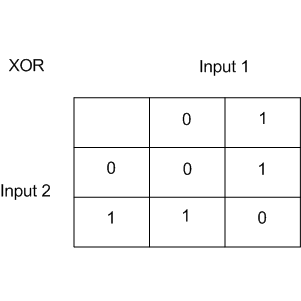XOR 是一个简单的二元分类问题，其中两个分类呈对角交叉分布。下面创建数据集。

In :
rng = np.random.RandomState(0)
X = rng.randn(200, 2)
y = np.logical_xor(X[:, 0] > 0, X[:, 1] > 0)

In :
plt.scatter(X[:, 0], X[:, 1], s=30, c=y, cmap=plt.cm.Paired)

Out:
<matplotlib.collections.PathCollection at 0x7fd9033288d0>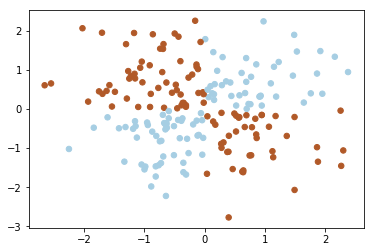In :
def plot_boundary(clf, X, y, plot_title):
xx, yy = np.meshgrid(np.linspace(-3, 3, 50), np.linspace(-3, 3, 50))
clf.fit(X, y)
# plot the decision function for each datapoint on the grid
Z = clf.predict_proba(np.vstack((xx.ravel(), yy.ravel())).T)[:, 1]
Z = Z.reshape(xx.shape)

image = plt.imshow(
Z,
interpolation="nearest",
extent=(xx.min(), xx.max(), yy.min(), yy.max()),
aspect="auto",
origin="lower",
cmap=plt.cm.PuOr_r,
)
contours = plt.contour(xx, yy, Z, levels=, linewidths=2, linetypes="--")
plt.scatter(X[:, 0], X[:, 1], s=30, c=y, cmap=plt.cm.Paired)
plt.xticks(())
plt.yticks(())
plt.xlabel(r"$x_1$")
plt.ylabel(r"$x_2$")
plt.axis([-3, 3, -3, 3])
plt.colorbar(image)
plt.title(plot_title, fontsize=12)

In :
plot_boundary(
LogisticRegression(solver="lbfgs"), X, y, "Logistic Regression, XOR problem"
)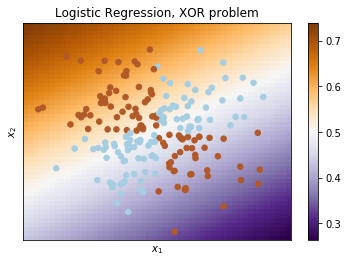In :
from sklearn.pipeline import Pipeline
from sklearn.preprocessing import PolynomialFeatures

In :
logit_pipe = Pipeline(
[
("poly", PolynomialFeatures(degree=2)),
("logit", LogisticRegression(solver="lbfgs")),
]
)

In :
plot_boundary(logit_pipe, X, y, "Logistic Regression + quadratic features. XOR problem")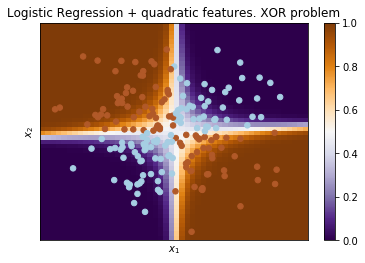### 验证和学习曲线¶

In :
import numpy as np
import pandas as pd
from matplotlib import pyplot as plt
from sklearn.linear_model import (LogisticRegression, LogisticRegressionCV,
SGDClassifier)
from sklearn.model_selection import learning_curve, validation_curve
from sklearn.pipeline import Pipeline
from sklearn.preprocessing import PolynomialFeatures, StandardScaler


• 应该让模型更复杂还是更简单？
• 应该加入更多特征吗？
• 是否只是需要更多数据用于训练？

In :
data = pd.read_csv("../../data/telecom_churn.csv").drop("State", axis=1)
data["International plan"] = data["International plan"].map({"Yes": 1, "No": 0})
data["Voice mail plan"] = data["Voice mail plan"].map({"Yes": 1, "No": 0})

y = data["Churn"].astype("int").values
X = data.drop("Churn", axis=1).values


In :
alphas = np.logspace(-2, 0, 20)
sgd_logit = SGDClassifier(loss="log", n_jobs=-1, random_state=17, max_iter=5)
logit_pipe = Pipeline(
[
("scaler", StandardScaler()),
("poly", PolynomialFeatures(degree=2)),
("sgd_logit", sgd_logit),
]
)
val_train, val_test = validation_curve(
logit_pipe, X, y, "sgd_logit__alpha", alphas, cv=5, scoring="roc_auc"
)


In :
def plot_with_err(x, data, **kwargs):
mu, std = data.mean(1), data.std(1)
lines = plt.plot(x, mu, "-", **kwargs)
plt.fill_between(
x,
mu - std,
mu + std,
edgecolor="none",
facecolor=lines.get_color(),
alpha=0.2,
)

plot_with_err(alphas, val_train, label="training scores")
plot_with_err(alphas, val_test, label="validation scores")
plt.xlabel(r"$\alpha$")
plt.ylabel("ROC AUC")
plt.legend()
plt.grid(True)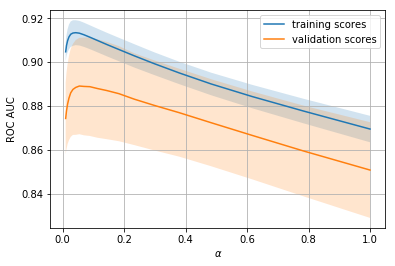• 简单模型的训练误差和验证误差很接近，且都比较大。这暗示模型欠拟合，参数数量不够多。
• 高度复杂模型的训练误差和验证误差相差很大，这暗示模型过拟合。当参数数量过多或者正则化不够严格时，算法可能被数据中的噪声「转移注意力」，没能把握数据的整体趋势。

### 数据对于模型的影响¶

In :
def plot_learning_curve(degree=2, alpha=0.01):
train_sizes = np.linspace(0.05, 1, 20)
logit_pipe = Pipeline(
[
("scaler", StandardScaler()),
("poly", PolynomialFeatures(degree=degree)),
(
"sgd_logit",
SGDClassifier(n_jobs=-1, random_state=17, alpha=alpha, max_iter=5),
),
]
)
N_train, val_train, val_test = learning_curve(
logit_pipe, X, y, train_sizes=train_sizes, cv=5, scoring="roc_auc"
)
plot_with_err(N_train, val_train, label="training scores")
plot_with_err(N_train, val_test, label="validation scores")
plt.xlabel("Training Set Size")
plt.ylabel("AUC")
plt.legend()
plt.grid(True)


In :
plot_learning_curve(degree=2, alpha=10)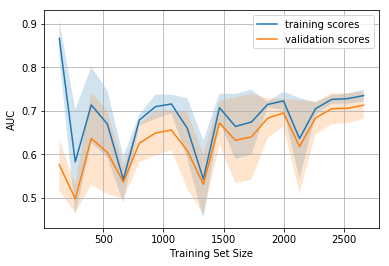In :
plot_learning_curve(degree=2, alpha=0.05)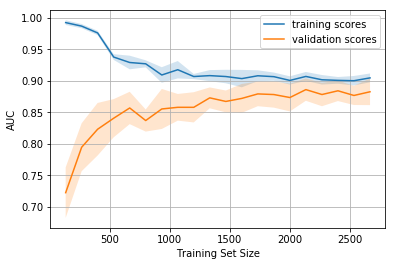In :
plot_learning_curve(degree=2, alpha=1e-4)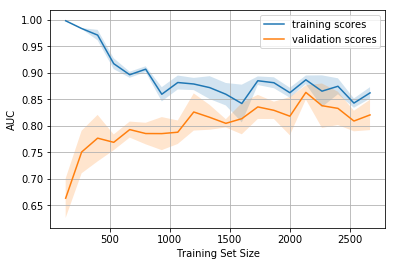• 训练集上的误差本身不能说明模型的质量。

• 交叉验证误差除了可以显示模型对数据的拟合程度外，还可以显示模型保留了多少对新数据的概括能力。

• 验证曲线是一条根据模型复杂度显示训练集和验证集结果的曲线：如果两条曲线彼此接近，且两者的误差都很大，这标志着欠拟合；如果两条曲线彼此距离很远，这标志着过拟合。
• 学习曲线是一个根据观测数量显示训练集和验证集结果的曲线：如果两条曲线收敛，那么增加新数据收益不大，有必要改变模型复杂度；如果两条曲线没有收敛，增加新数据可以改善结果。